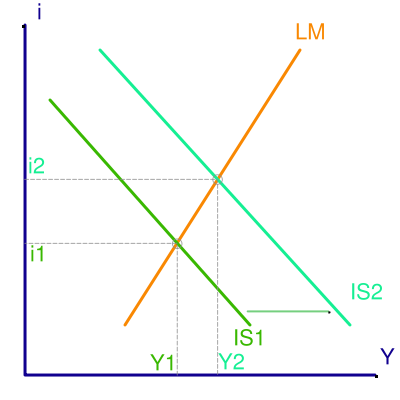xCrowding out (economics)EncyclopediaIn economics, crowding out occurs when Expansionary Fiscal Policy causes interest rates to rise, thereby reducing private spending. That means increase in government spending crowds out investment spending.

Changes in fiscal policy shifts IS curve, the curve describes equilibrium in the goods market. A Fiscal Expansion shifts IS curve to the right from IS1 to IS2. A fiscal expansion increases equilibrium income from Y1 to Y2 and interest rates from i1 to i2. At unchanged interest rates i1, the higher level of government spending increase the level of Aggregate Demand
Aggregate demand
In macroeconomics, aggregate demand is the total demand for final goods and services in the economy at a given time and price level. It is the amount of goods and services in the economy that will be purchased at all possible price levels. This is the demand for the gross domestic product of a...

. This increase in demand must be met by rise in output. At each level of interest rate, equilibrim income must rise by the multiplier
Multiplier
The term multiplier may refer to:In electrical engineering:* Binary multiplier, a digital circuit to perform rapid multiplication of two numbers in binary representation* Analog multiplier, a device that multiplies two analog signals...

times the increase in government spending.

If the interest rate stayed constant at i1, the goods market is in equilibrium in that planned spending equals output. But the assets market is no longer in equilibrium. Income has increased and therefore the quantity of money demanded is higher. Becaused there is an excess demand for real balances, the interest rate rises. Firms planned spending declines at higher interest rates, thus the aggregate demand falls. Therefore the equilibrium is at higher interest rates. The adjustment of interest rates and their impact on aggregate demand dampen the expansionary effect of the increased government spending.

## What factors determine how much crowding out takes place ?

The extend to which interest rate adjustments dampen the output expansion induced by increased government spending is determined by -
(a) Income increases more, interest rates increase less, the flatter LM curve.
(b) Income increases less, interest rates increase less, the flatter IS curve.
(c) Income and interest rates increase more the larger the multiplier, thus, the larger the horizontal shift in the IS curve.
In each case, the extend of crowding out is greater the more interest rate increases when government spending rises.

### The Liquidity Trap

If the economy is in the liquidity trap, the LM curve is horizontal, an increase in government spending has its full multiplier effect on the equilibrium income. There is no change in the interest associated with the change in government spending, thus no investment spending cut off. Therefore no dampening of the effects of increased government spending on income.
If the demand for money is very sensitive to interest rates, so that the LM curve is almost horizontal, fiscal policy changes have a relatively large effect on output, while monetary policy changes have little effect on the equilibrium output. So, if the LM curve is horizontal, monetary policy has no impact on the equilibrium of the economy and the fiscal policy has a maximal effect.

### The Classical Case and Crowding Out

If the LM curve is vertical, then an increase in government spending has no effect on the equilibrium income and only increases the interest rates. If the demand for money is not related to the interest rate, as the vertical LM curve implies, then there is unique level of income at which the money market is in equilibrium.

Thus, with vertical LM curve, an increase in government spending cannot change the equilibrium income and only raises the equilibrium interest rates. But if government spending is higher and the output is unchanged, there must be an offsetting reduction in private spending. In this case, the increase in interest rates crowds out an amount of private spending equal to increase in government spending. Thus, there is full crowding out if LM is vertical.

## Is Crowding Out Likely?

The question is how seriously we must take the possibility of crowding out? This is discussed in three points given below.

### First

The first point is rather an important warning.When we consider an economy with prices given, in which output is below the full employment level. In such conditions, when fiscal expansion increases demand, firms can increase the output by hiring more workers. But when the economy is at full employment level, crowding out becomes a much more realistic possibility because firms cannot then increase their output. In this condition an increase in demand will lead to an increase in price level rather than an increase in output.

### Second

In an economy with unemployed resources there will not be full crowding out because LM curve is not vertical. A fiscal expansion will raise interest rates, but income will also rise. Crowding out, thus, is a matter of degree.

### Third

With unemployment in the economy, there is possibility for output to expand, interest rates will not rise at all when government spending rises and there not be any crowding out. This is true because the monetary authorities can accommodate the fiscal expansion by an increase in the money supply, thus preventing interest rates to rise. This monetary accomodation is referred to as Monetizing Budget Deficits, meaning central bank prints more money to buy the bonds with which the government pays for its deficit. In this case, both IS - LM curve shift to right, increasing output at same interest rate. Accordingly, there need not be any adverse effects on investment.
The source of this article is wikipedia, the free encyclopedia.  The text of this article is licensed under the GFDL.